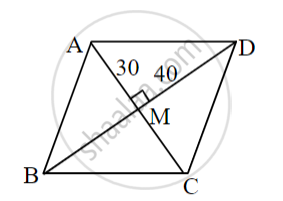# Choose the correct alternative: If length of both diagonals of rhombus are 60 and 80, then what is the length of side? - Geometry

MCQ

Choose the correct alternative:

If length of both diagonals of rhombus are 60 and 80, then what is the length of side?

• 100

• 50

• 200

• 400

#### Solution

50Let ABCD be the rhombus, diagonal AC = 60 and BD = 80

we know that the diagonals of a rhombus are perpendicular bisectors of each other.

∴ Diagonals AC and BD bisect each other at point M.

∴ In ∆AMD, ∠M = 90°, AM = 30, DM = 40

∴ AM2 + DM2 = AD2   ...[Pythagoras theorem]

∴ (30)2 + (40)2 = AD2

∴ 900 + 1600 = AD2